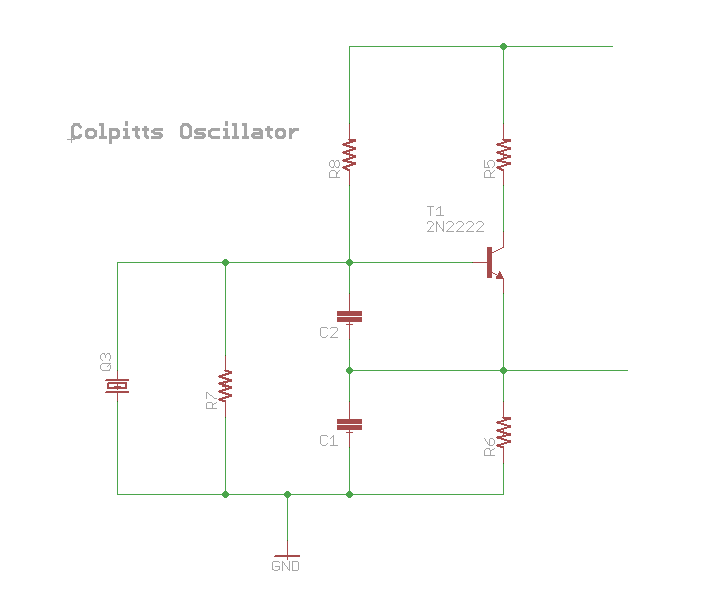# Oscillator

Sometimes we need a timed signal to use as a clock (but also for other things).

## General theory

The Barkhausen stability criterion says that

1. the loop gain exceeds unity at the resonant frequency
2. the fase shift around the loop is $2\pi n$ (where $n\in N$)

bad enough seems that the Barkhausen Stability Criterion is simple, intuitive, and wrong.

## Crystal

Quartz is a piezoelectric material. When an electric field is placed upon it, a physical displacement occurs. Interestingly enough, we can write an equivalent electrical circuit to represent the mechanical properties of the crystal.

## Colpitts oscillator• slide with derivation formulas for the colpitts oscillator
• Video Colpitts Crystal Oscillator Fundamentals
• http://www.electronics-tutorials.ws/oscillator/colpitts.html
• http://www.electronics-tutorials.ws/oscillator/crystal.html
• https://makerf.com/posts/experimenting_with_colpitts_oscillators
• Crystal Oscillator Design with video and web app to calculate stuffs

## Negative resistance oscillator

Some active devices (like diode and transistor) have zone of negative resistance that can be used to amplify the feedback loop of an oscillating circuit.

• Video World's Simplest Single Transistor Oscillator - BJT with Negative Resistance
• Page with a more elaborated explanation of NR.

## Voltage controlled oscillator (VCO)

• https://www.electronicshub.org/voltage-controlled-oscillators-vco/
• https://electronics.stackexchange.com/questions/233607/sawtooth-vco-and-current-source-for-modular-synthesizer
• Posts (1 2 e 3) about building a VCO creating a current source
• https://electronics.stackexchange.com/questions/207668/how-do-i-make-a-vco-with-a-lm-358